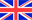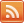Latest NewsCross+ASudoku

Sudoku (also known as "Number Place") is a placement puzzle. The puzzle is most frequently a 9 x 9 grid made up of 3 x 3 subgrids (called "regions"). Some cells already contain numbers, known as "givens". The goal is to fill in the empty cells, one number in each, so that each column, row, and region contains the numbers 1 through 9 exactly once. Each number in the solution therefore occurs only once in each of three "directions", hence the "single numbers" implied by the puzzle's name.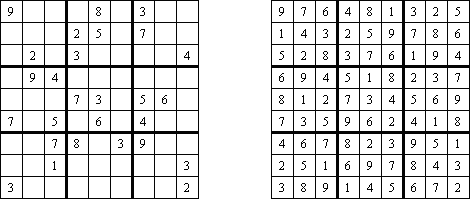Cross+A can solve and create many kinds of sudokus.

In some sudokus main diagonals also contain the digits 1 through 9. This variant is called Sudoku X ("Diagonal Sudoku"). Use the check box Diagonals for such sudokus.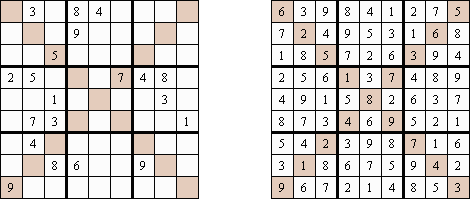Argyle Sudoku contains additional diagonals; every marked diagonal line contains each digit not more than once. The argyle pattern is derived from the tartan of Clan Campbell, in eastern Scotland.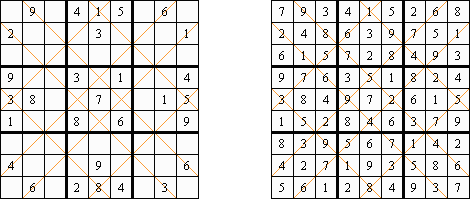Also the puzzle may contain the regions of random (irregular) shape; this variant is called Jigsaw Sudoku ("Geometry Sudoku", "Geometry Number Place", "Irregular Sudoku", "Kikagaku Nanpure").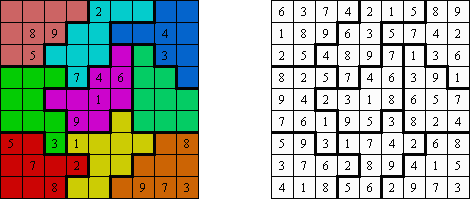Godoku (also known as "Wordoku", "Alphabet Sudoku") is similar to sudoku, but uses letters instead of numbers. Once the grid is complete, the hidden word may be revealed.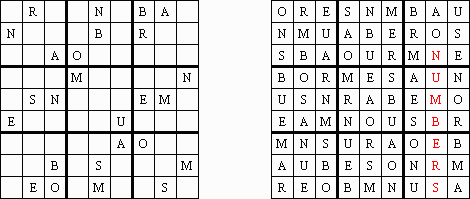In Asterisk Sudoku there is an additional area, shown on the grid by shading nine specially selected cells. Those nine cells must also contain 1 to 9.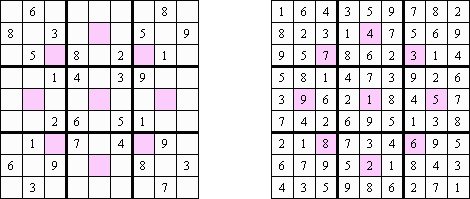Girandola is an ordinary sudoku with one extra group of cells. This group must also contain digits 1 through 9. (Girandola is a kind of revolving firework.)Center Dot is a variant of sudoku, where central cells of each region form an extra region. This region must contain digits 1 through 9.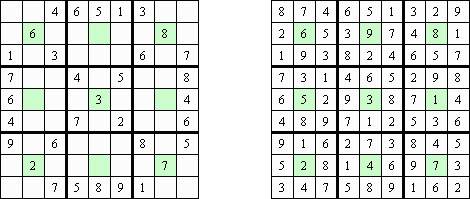Sudoku may contain additional four regions 3 x 3. This format was first introduced in the Dutch newspaper "NRC Handelsblad" by Peter Ritmeester. It is called Windoku ("Four-Box Sudoku", "Hyper Sudoku").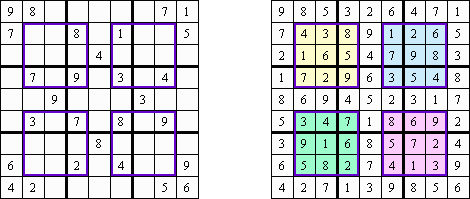Sudoku-DG ("Offset Sudoku") contains 9 disjoint groups in the puzzle, one for each relative box position. Each group has a distinct color. The group of 9 cells with the same color must also contain digits 1 through 9.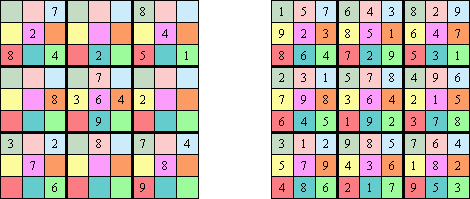In Anti-Knight Sudoku all cells at a chess knight move (at a distance of 2 by 1) must hold different numbers.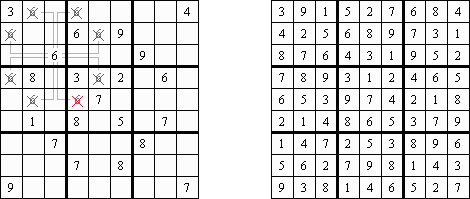In Anti-King Sudoku ("Touchless Sudoku") equal digits can be neither orthogonally nor diagonally adjacent.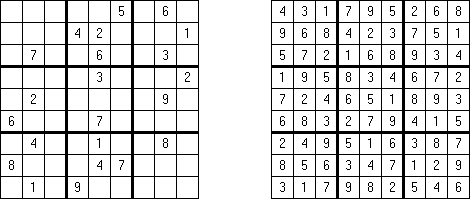In Anti Diagonal Sudoku each diagonal has at most three different digits.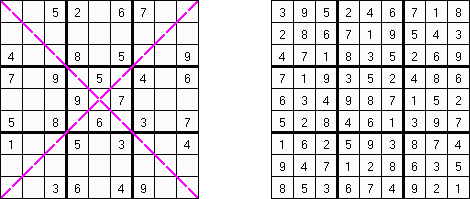In Killer Sudoku, sub-regions have specified sums, and no number can be repeated within a region. This variant also called "Sums Sudoku", "Sums Number Place", "Samunamupure", "Kikagaku Nampure".Greater Than Sudoku is the variation of the original sudoku. In addition, greater than or less than signs (">" or "<") in the walls indicate that one cell's number is greater than or less than an adjacent cell's number. Also called "Compdoku".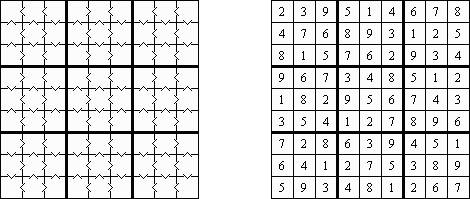Even-Odd Sudoku: fill in the grid so that every row, every column, and every 3 x 3 box contains the digits 1 through 9. Cells with gray circles must contain odd digits, cells with gray squares must contain even digits.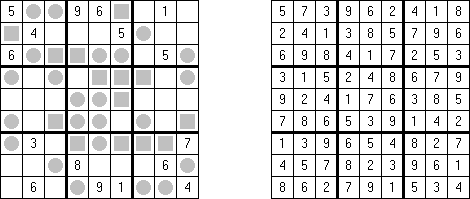In Consecutive Sudoku ("1-away Disallowed Number Place"), all the places where orthogonally adjacent cells are consecutive numbers have been specially marked.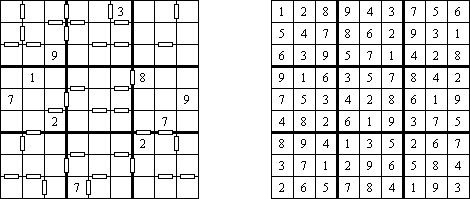In Non-Consecutive Sudoku no two adjacent cells have consecutive values. For example, if a cell contains 3, then any neighbouring square cannot contain either 2 or 4.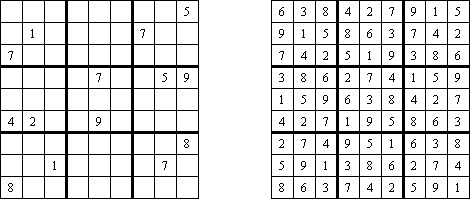Kropki Sudoku (the Polish word "kropki" means "dots"; also known as "Dots Sudoku") is the variation of the original sudoku. If absolute difference between two digits in neighboring cells equals 1, then they are separated by a white dot. If the digit is a half of digit in the neighboring cell, then they are separated by black dot. The dot between 1 and 2 can be either white or black.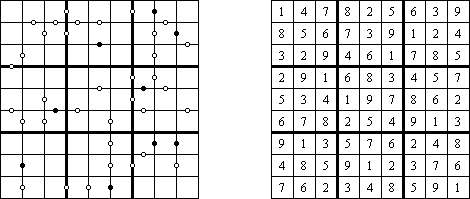Sukaku ("Suuji Kakure", "Pencilmark Sudoku") has 81 groups of digits in a 9 x 9 grid. Like a standard sudoku, the puzzle must be completed in such a way that each row, each column and each 3 x 3 box contains digits 1 through 9. Only the candidates shown in the grid can be used.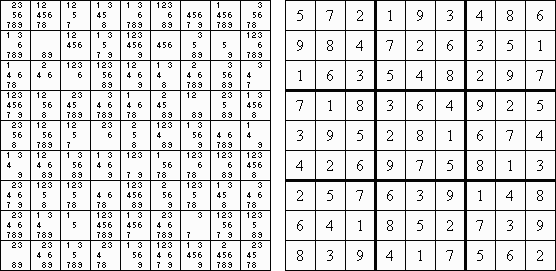Chain Sudoku (also known as "Strimko") consists of a group of circles arranged in a square grid and containing given clues in various places. The object is to fill all empty circles so that the digits appear exactly once in each row, column and chain.

The program can solve and create puzzles from 4 x 4 to 9 x 9.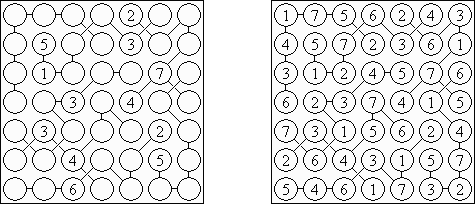Frame Sudoku ("Outside Sum Sudoku") consists of an empty grid. Numbers in the outside frame equal the sum of the first three numbers in the corresponding row or column in the given direction.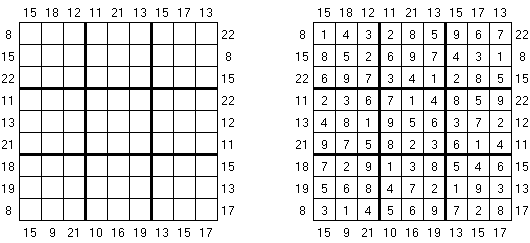Skyscraper Sudoku contains clues around the edge. The task is to place digits in the grid. Each digit represents the height of the skyscraper in each cell. The numbers outside the grid represent the number of skyscrapers seen from the corresponding direction.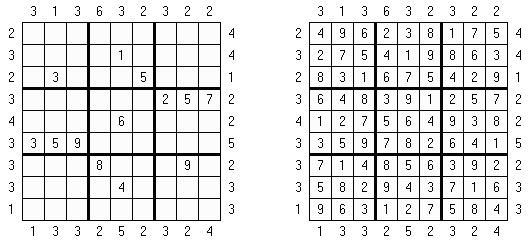Tripod Sudoku is a variation of sudoku, where the borders of regions are not defined. The goal is to fill the grid with digits and divide the grid into regions. Each digit appears exactly once in every row, column and region. All points where three lines meet are given. There are no points where four lines meet.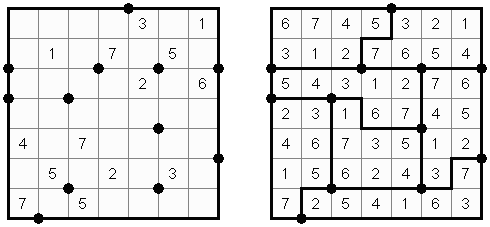Sudoku Mine combines Sudoku puzzle with Minesweeper game. The goal is to place three mines in every row, column and region. The digits in the grid represent the number of mines in the neighbouring cells.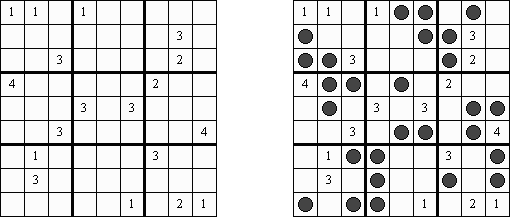Sujiken (from Japanese "sujikai", literally "diagonal") is a variation of Sudoku, invented by George Heineman (USA). The puzzle consists of a triangular grid of cells containing digits from 1 to 9. The objective is to fill a grid with digits so that each cell contains a digit and no digit is repeated in any column, row and diagonal in any direction. Also, no digit occurs twice in any of the three larger 3 x 3 square regions and any of the three larger triangular regions enclosed by thick borders.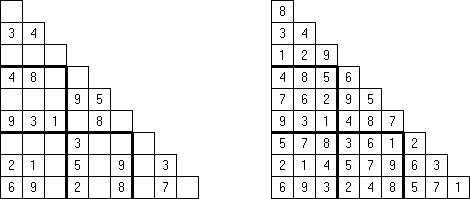Sudoku XV is the variation of the original sudoku. All adjacent cells with two digits summing to 10 are marked by X, while those summing to 5 are marked by V. The cells edges which do not contain an X or a V cannot have digits summing to 5 or 10.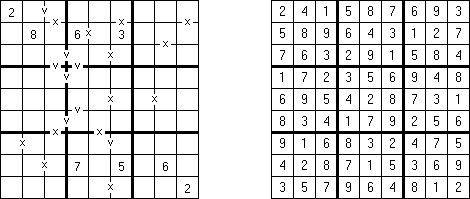Outside Sudoku is a variation of the standard sudoku. Some digits are given outside the grid. These digits must appear in the first three cells in the corresponding direction.Rossini Sudoku is a variation of the classic sudoku. The arrows outside the grid indicate that the nearest three digits in the corresponding direction are in ascending or descending order (the highest number is always in the direction of the arrow). All possible arrows are given, so if there is no arrow, the first three digits do not form an increasing sequence in either direction.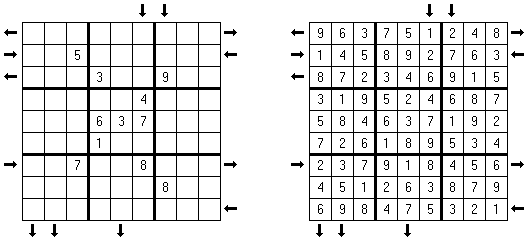Little Killer Sudoku contains numbers and arrows around the edge. The numbered arrows outside the grid indicate the sum of the digits in the corresponding direction. Digits can repeat in the direction of the arrow.Little Unique Killer Sudoku is a variation of Little Killer Sudoku. Clues outside the grid indicate the sums of digits in the direction of the corresponding arrow. Digits may not repeat within a sum.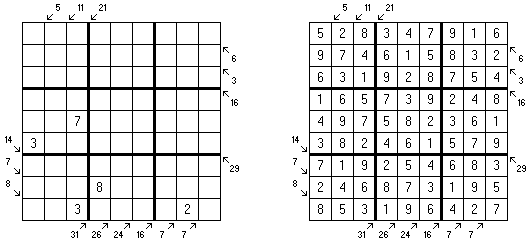Vudoku is a variation of the classic sudoku. The digit at the vertex of each marked "V" must either be sum or difference of other two digits on the "V".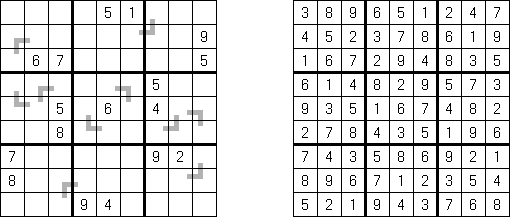Sandwich Sudoku contains extra numbers outside the grid. These extra clues indicate the sum of digits between 1 and 9 in the corresponding row or column.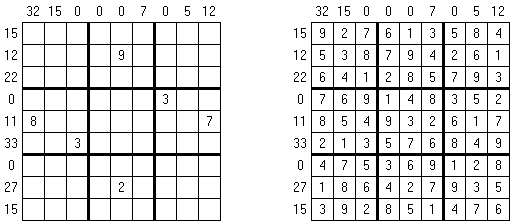The program can solve puzzles of different grid sizes:

• 4 x 4 (the subgrids' size is 2 x 2);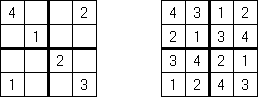• 6 x 6 (the subgrids' size is 3 x 2);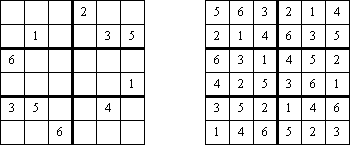• 8 x 8 (the subgrids' size is 4 x 2);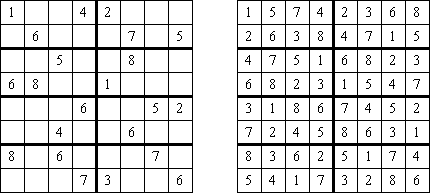• 10 x 10 (the subgrids' size is 5 x 2);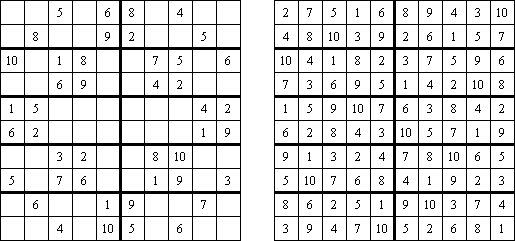• 12 x 12 (the subgrids' size is 4 x 3);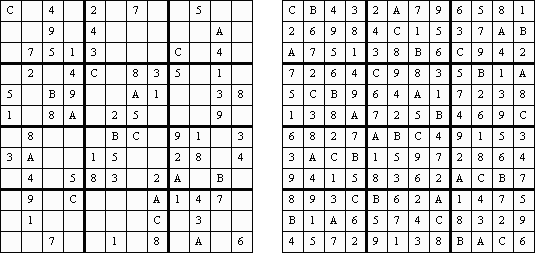• 15 x 15 (the subgrids' size is 5 x 3);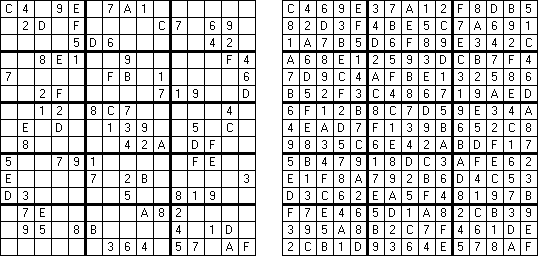• 16 x 16 (the subgrids' size is 4 x 4).Gattai is a Japanese word meaning merged or fused; this term is used for overlapping sudoku. A number after the word "Gattai" indicates how many 9 x 9 sudokus are in the puzzle ("Gattai-3", "Gattai-4", "Gattai-5" etc.).

Samurai Sudoku ("Gattai-5") is one of the sudoku variation with overlapping sudoku grids. The puzzle consists of five grids, one in the center and the other 4 overlapping each corner grid of the central one. This means that the numbers must be placed correctly for all the five puzzle squares.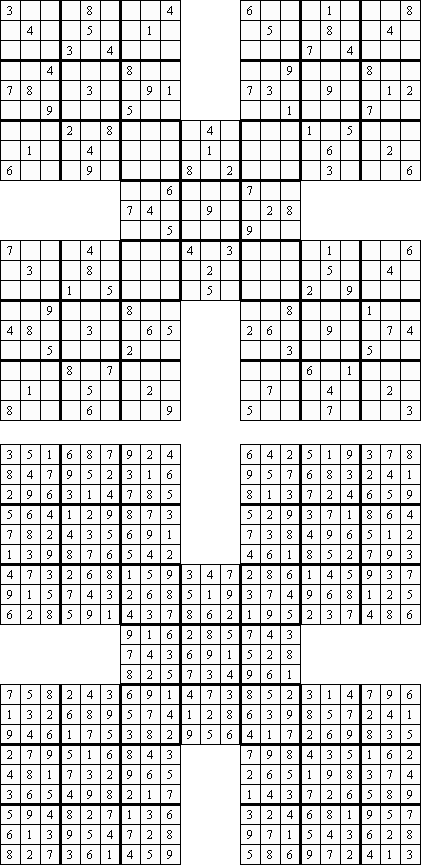Flower Sudoku ("Musketry Sudoku") has five sudoku puzzles in layout, similar to Samurai Sudoku. However, these puzzles overlap each other much more than in Samurai. The center grid is fully covered by the remaining four sub-puzzles.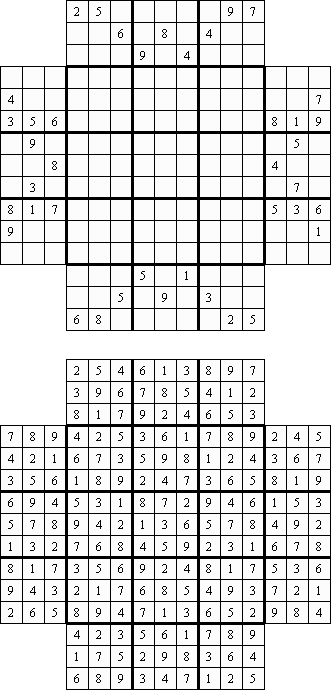Named for the warrior monks of medieval Japan, Sohei Sudoku has four merged grids. Each grid has two overlapping areas.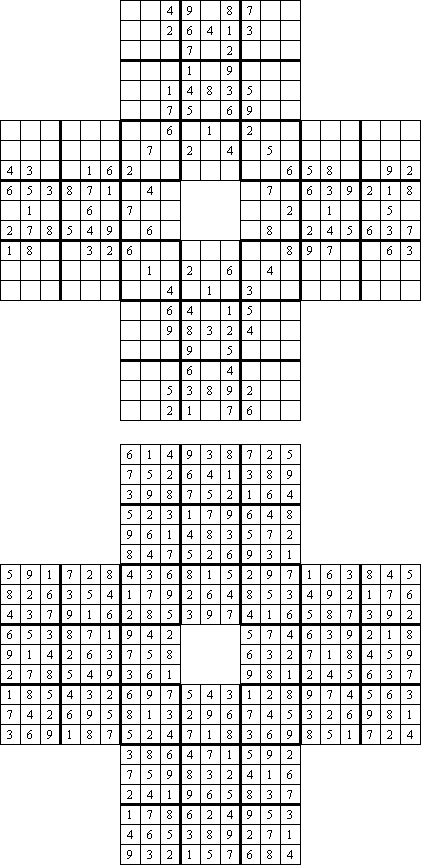Kazaguruma ("Windmill Sudoku") consists of five grids, one in the center and the other 4 overlapping the central one. This means that the numbers must be placed correctly for all the five puzzle squares.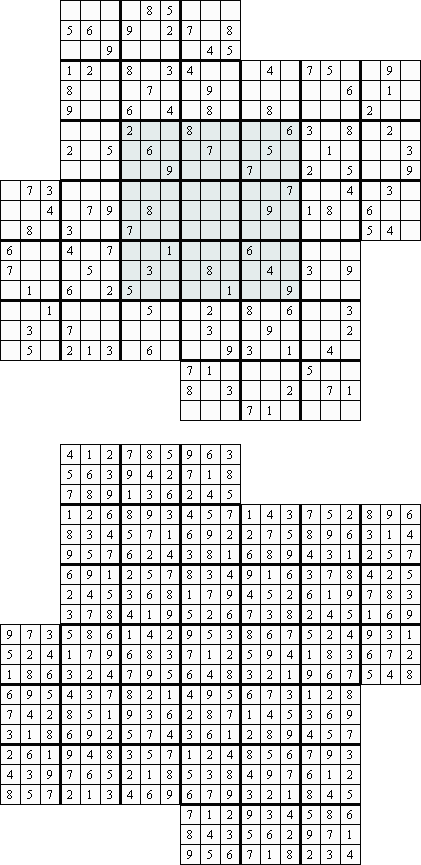Butterfly Sudoku is the variation of the original sudoku. The puzzle consists of four grids 9 x 9. The numbers must be placed correctly for all the four puzzle squares.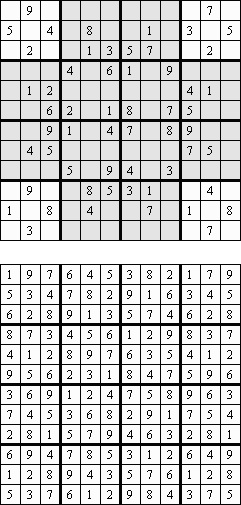Cross Sudoku has five merged grids. The numbers must be placed correctly for all the five puzzle squares.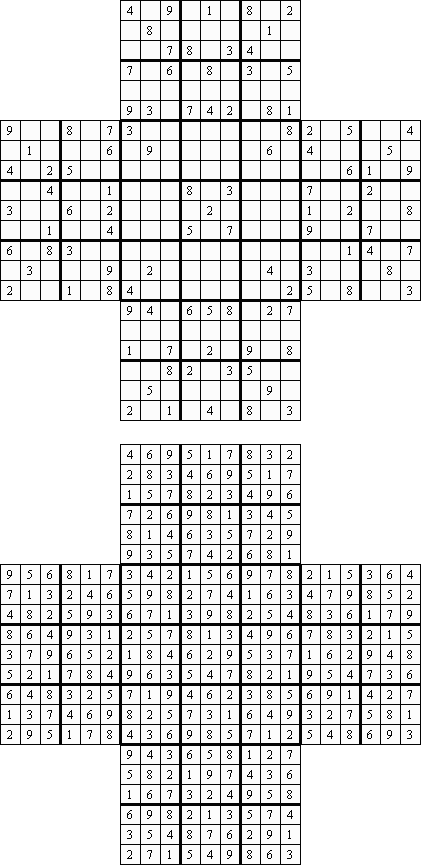Gattai-3 consists of three grids 9 x 9.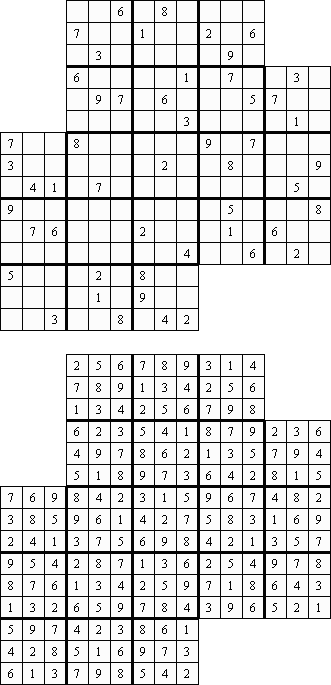Twodoku ("Sensei Sudoku", "DoubleDoku") consists of two grids 9 x 9. The numbers must be placed correctly for both puzzle squares.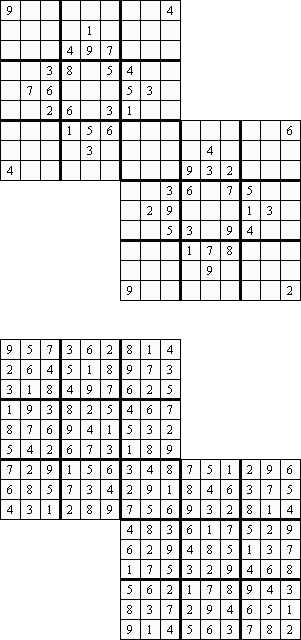The program can solve Twodoku with irregular regions: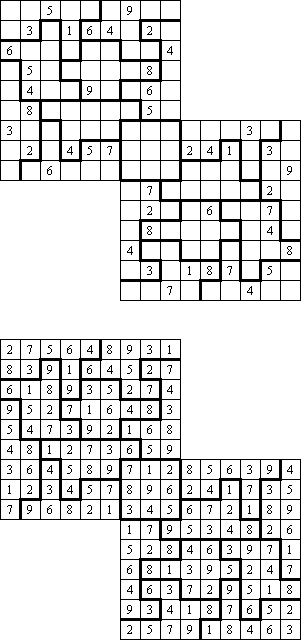Triple Doku consists of three grids 9 x 9. The numbers must be placed correctly for all puzzle squares.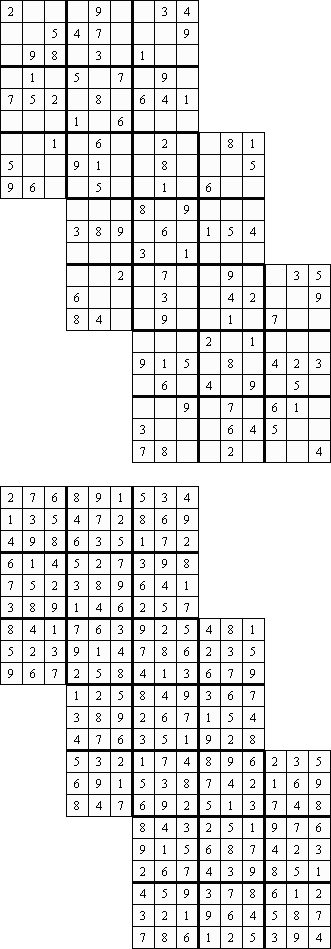Twin Corresponding Sudoku consists of a pair of standard sudoku puzzles each with some starting digits. To get a complete solution for the twin puzzles, it is necessary to solve each twin puzzle, but the complete solution of the twin puzzles will be obtained by substituting the corresponding values of the digits from one twin sudoku into the other.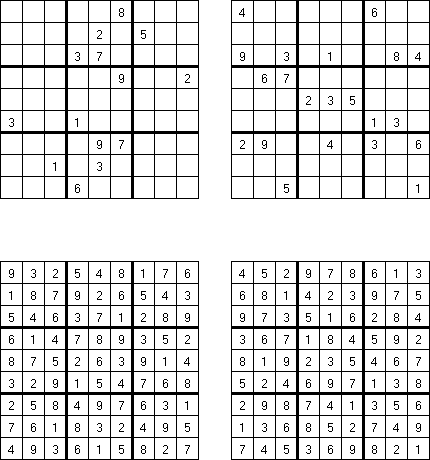Hoshi consists of six large triangles; the numbers 1 through 9 must be placed into the triangular cells of each large triangle. Every line (of any length, even uncontinous) contains every digit not more than once.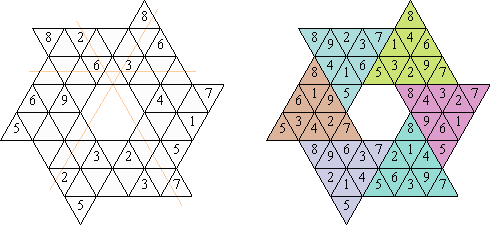In Star Sudoku the row on the outer edge of the grid includes the cell on the nearest sharp end of the figure.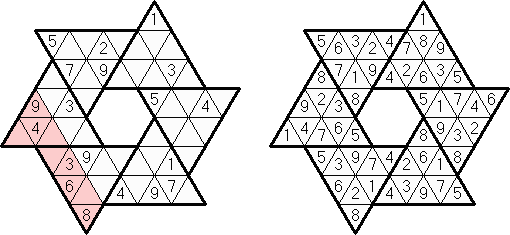Tridoku was invented by Japheth Light (USA). The puzzle consists of nine large triangles; the numbers 1 through 9 must be placed into the triangular cells of each large triangle. Also, the numbers 1 through 9 must be placed in the three legs of the inner shaded triangle and in the three legs of the outer shaded triangle. No two neighboring cells may contain the same number.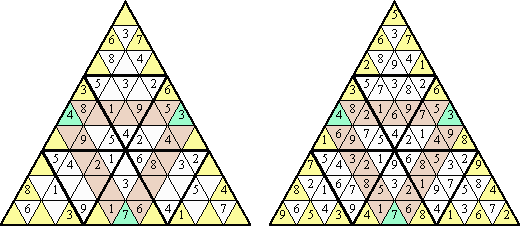Sudoku Variants and other puzzles
A list of Sudoku variations, collected by Uwe Wiedemann, with descriptions and examples.